Vikas Verma — April 15, 2021
This article was published as a part of the Data Science Blogathon.

## Introduction

In today’s world, every second the data keeps on getting bigger and bigger. In order to understand the data quickly and to draw insights, data visualization becomes necessary.

For e.g. consider a case where you are asked to illustrate crucial sales aspects (like sales performance, target, revenue, acquisition cost, etc.) from huge amounts of sales data, which one would you prefer:

1. Exploring the data using Excel (or spreadsheets) and keeping track of each sales aspect manually.
2. Exploring the data using different types of sales graphs and charts

Obviously, you would prefer graphs and charts. So data visualization plays a key role in data exploration and data analysis.

Data Visualization is the technique to represent the data/information in a pictorial or graphical format. It enables the stakeholders and decision-makers to analyze and explore the data visually and uncover deep insights.

“Visualization gives you answers to questions you didn’t know you had.” – Ben Schneiderman

## Benefits of Data Visualization

• Helps in data analysis, data exploration and makes the data more understandable.
• Summarises the complex quantitative information in a small space.
• Helps in discovering the latest trends, hidden patterns in the data.
• Identifies the relationships/correlations between the variables.
• Helps in examining the areas that need attention or improvement.

## Why Plotly?

There are several libraries available in Python like Matplotlib, Seaborn, etc. for data visualization. But they render only the static images of the charts/plots and due to this, many crucial things get lost in the visualization. Wouldn’t it be amazing if we could interact better with the charts by hovering in (or) zooming in? Plotly allows us to do the same.

• Plotly is an open-source data visualization library to create interactive and publication-quality charts/graphs.
• Plotly offers implementation of many different graph types/objects like line plot, scatter plot, area plot, histogram, box plot, bar plot, etc.
• Plotly supports interactive plotting in commonly used programming languages like Python, R, MATLAB, Javascript, etc.

In this post, we will cover the most commonly used graph types using Plotly. So let’s get started using the Cars93 dataset available on Kaggle.

The dataset contains 27 car parameters (like manufacturer, make, price, horsepower, engine size, weight, cylinders, airbags, passengers, etc.) of 93 different cars.

The dataset looks like this:

Additional NOTE: In order to access the entire python code follow the kaggle kernel here(https://www.kaggle.com/vikashrajluhaniwal/interactive-visualizations-using-plotly).

### Installing Plotly

In order to install Plotly, use the following command in the terminal.

`pip install plotly`

Plotly comes with few modules to create visualizations, i.e. giving us a choice of how to utilize it.

• express: A high-level interface for creating quick visualizations. It’s a wrapper around Plotly graph_objects module.
• graph_objects: A low-level interface to figures, traces, and layouts. It’s highly customizable in general for different graphs/charts.
• figure_factory: Figure Factories are dedicated functions for creating very specific types of plots. It was available prior to the existence of Plotly express, therefore deprecated as “legacy”.

Having known and installed Plotly, now let’s plot different graphs/charts using it.

### 1. Box Plot

• A box plot (or box-and-whisker plot) is a standardized way to display the distribution of quantitative data based on a Five-Point summary (minimum, first quartile(Q1), median(Q2), third quartile(Q3), and maximum).
• The box extends from the Q1 to Q3 quartile values, whereas the whiskers extend from the edges of the box to the 1.5*IQR. IQR = (Q3 – Q1)

Now let’s craft a box plot for cars’ Price feature.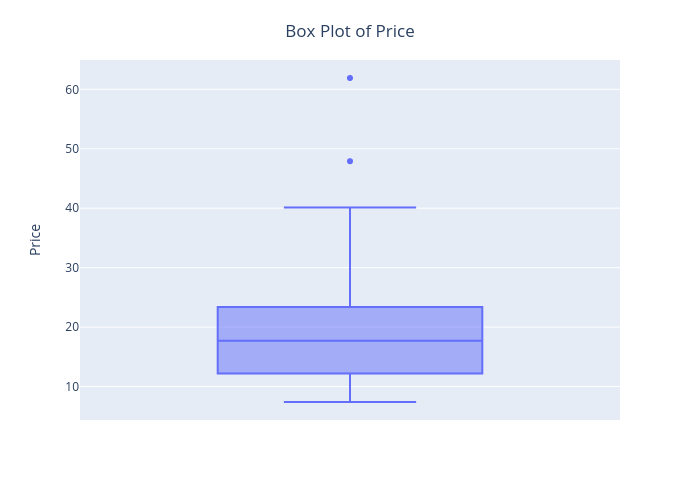The best thing about this visualization is that we can start interacting with it by hovering in to see the quantiles values.

Similarly, we can customize it as per the requirement. For e.g. drawing a boxplot of Price for each AirBags type.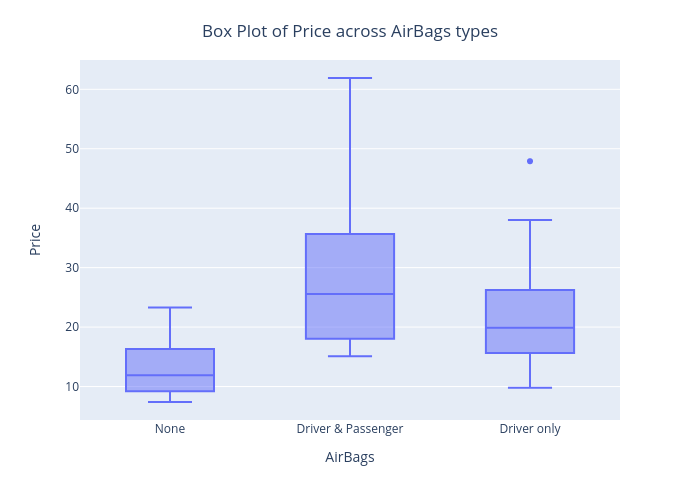2. Histogram

• A histogram is an accurate representation of the distribution of numerical data.
• To construct a histogram, follow these steps −
• Bin (or bucket) the range of values – Divide the entire range of values into a series of intervals.
• Count how many values fall into each interval.

Let’s draw a histogram for cars’ Horsepower feature.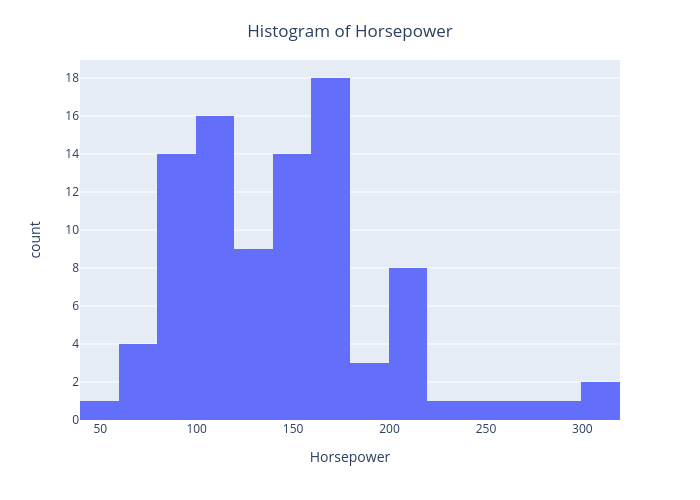Here, X-axis is about bin ranges of Horsepower whereas Y-axis talks about frequency/count in each bin.

### 3. Density Plot

• The density plot is a variation of a histogram, where instead of representing the frequency on the Y-axis, it represents the PDF (Probability Density Function) values.
• It’s helpful in determining the Skewness of the variable visually.
• Also, useful in assessing the importance of a continuous variable for a classification problem.

The density plot of Horsepower based on AirBags type is as shown below.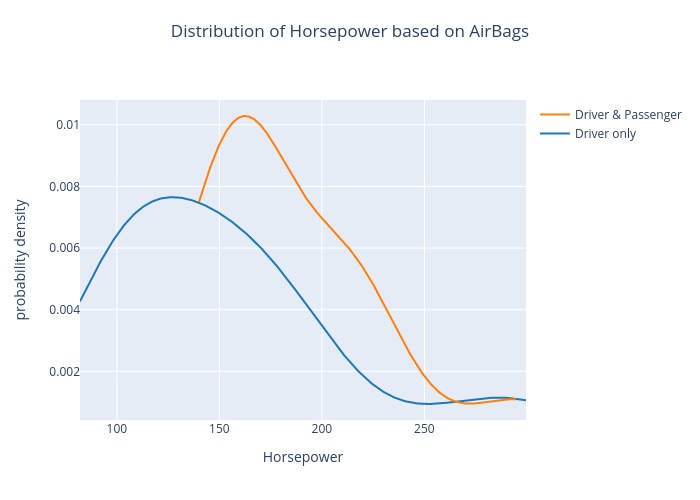### 4. Bar Chart

• A bar chart represents categorical data with rectangular bars with weights proportional to the values that they represent.
• A bar plot shows comparisons among discrete categories.

The bar chart of the Type feature is as shown below.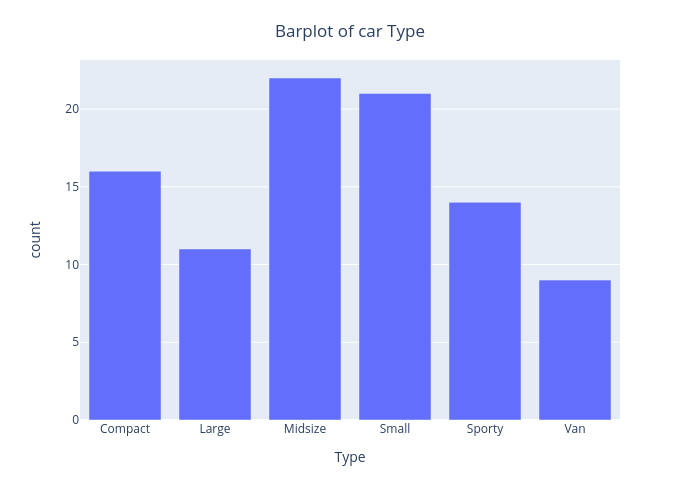Similarly, we can customize it to display MPG.city mean on the Y-axis, instead of displaying count.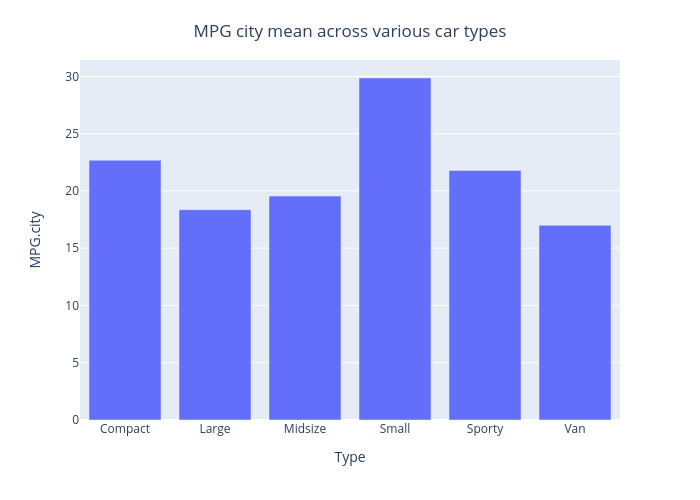### 5. Pie Chart

• Pie Chart is used to represent the numerical proportion of the data in a circular graph.
• The whole area of the chart represents 100% of the data, the arc length of each slice represents the relative percentage part of the whole.

The pie chart of the Type feature is as shown below.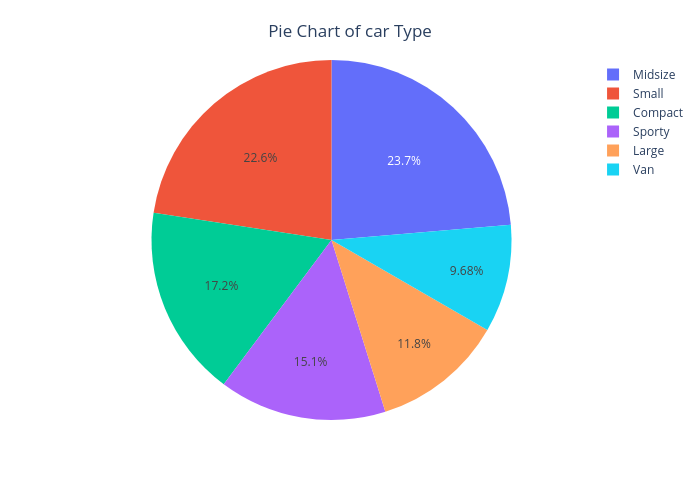### 6. Scatter Plot

• A scatter plot uses dots to represent values for two different numeric variables.
• It is really helpful in observing the relationship between two numeric variables.

Let’s draw a scatter plot, in order to assess the relationship between Horsepower and MPG.city.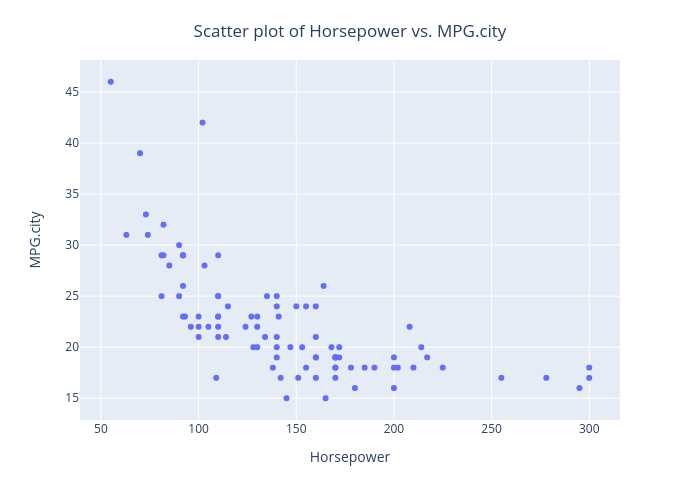From this plot, we can observe that as the Horsepower increases, MPG in the city decreases.

Plotly also provides a way to draw 3D scatter plots. Let’s draw the same using Horsepower, MPG.city, and Price features.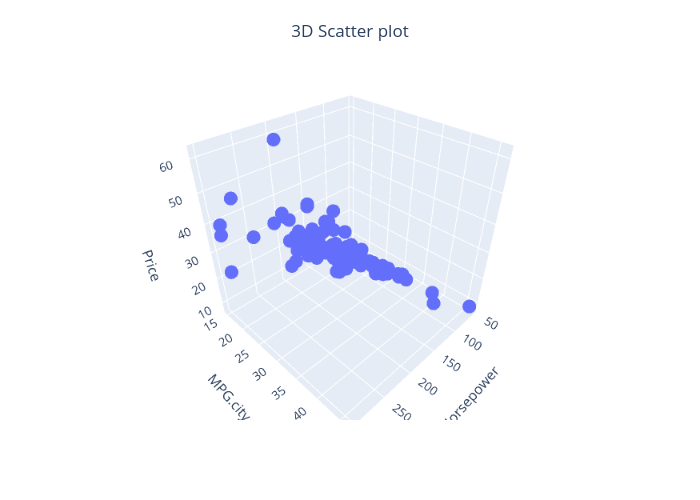Similarly, we can draw a scatter plot matrix (a grid/matrix of scatter plots) to assess pairwise relationships for each combination of variables.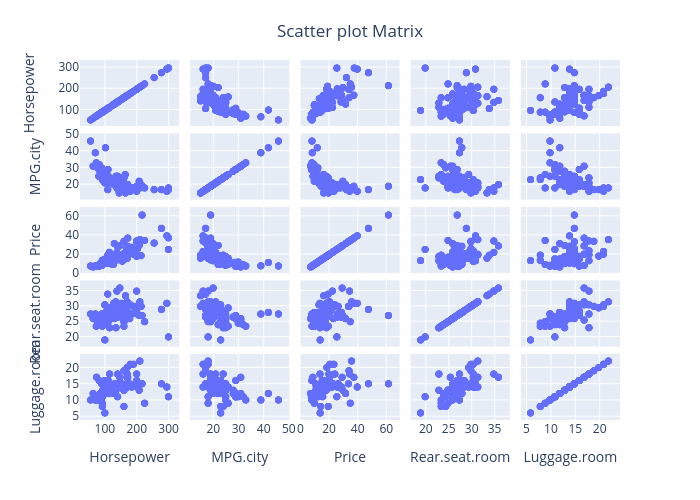### 7. Line Chart

• A line chart is a type of chart that displays information as a series of data points called ‘markers’ connected by straight line segments.
• It is similar to a scatter plot except that the measurement points are ordered (typically by their x-axis value) and joined with straight line segments.
• Line graphs are usually used to find relationships between two numeric variables or to visualize a trend in time series data.

Let’s draw a scatter plot, in order to assess the relationship between Horsepower and MPG.city.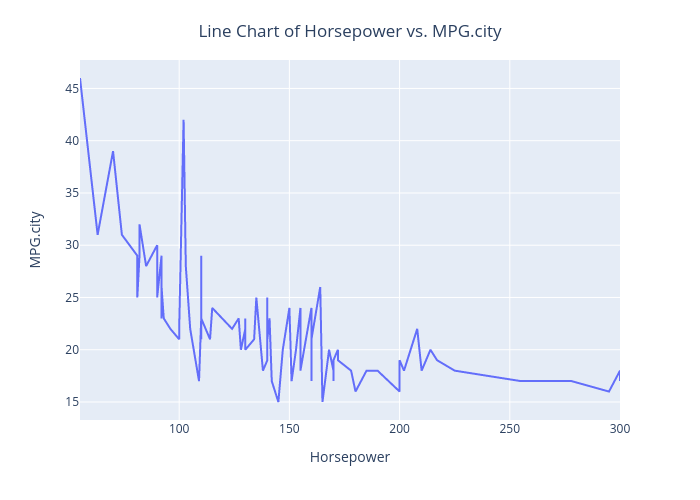### 8. Heatmap

• A heatmap is a two-dimensional graphical representation of data whereas matrix values are represented in different shades of colors.
• A heatmap aims to provide a color-coded visual summary of data/information.
• Seaborn allows annotated heatmaps as well.

Let’s draw a heatmap to represent the correlation matrix of cars93 data.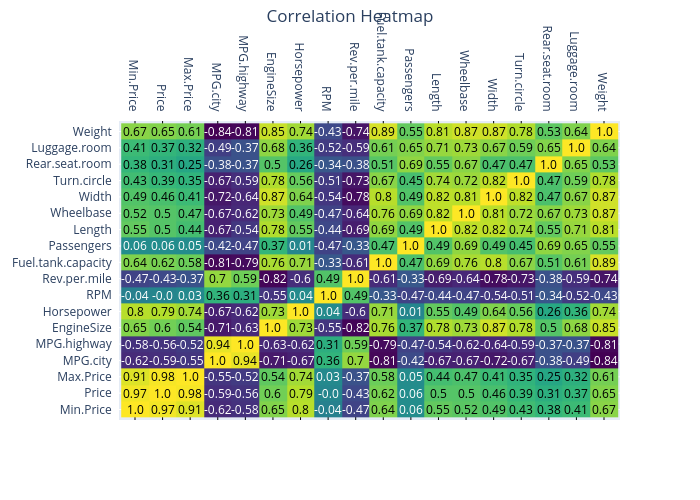### 9. Violin Plot

• Violin plots are similar to box plots, except that they also show the probability density of the data at different values. In other words, the violin plot is a combination of a box plot and density plot.
• Broader sections of the violin plot indicate higher probability, whereas the narrow sections indicate lower probability.

The Violin plot of the Price feature is shown below.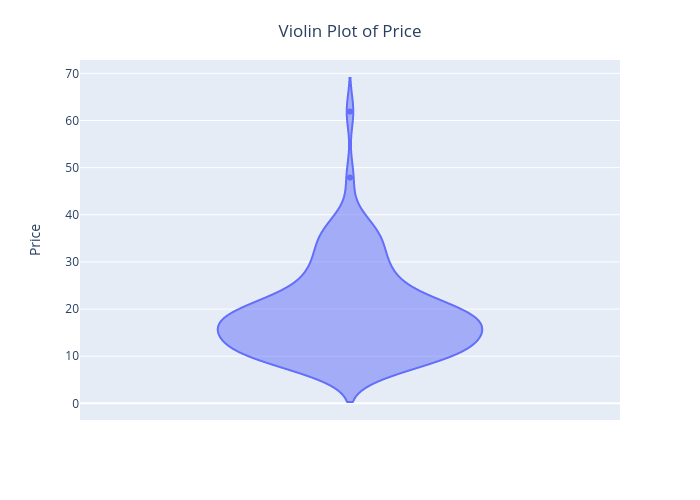Similarly, we can customize it using Plotly to display the box and all the data points.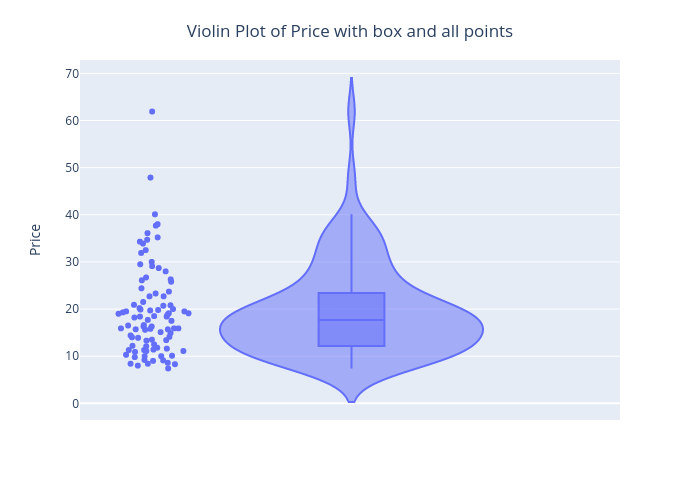### 10. Word Cloud

• Word Cloud is a visualization technique to represent the frequency of words within a given text segment.
• The size of a word indicates how frequently it occurs in the text. The bigger the size, the greater the importance(frequency), whereas the smaller the size, the lesser the importance(frequency).
• Word clouds are often used for representing the frequency of words within text documents, reports, website data, public speeches, etc.

Word cloud of a chosen text document is as shown below.

## End Notes

In this article, we discussed different types of plots/charts using Plotly in Python. Plotly is highly recommended for creating interactive visualizations.

The media shown in this article are not owned by Analytics Vidhya and is used at the Author’s discretion.